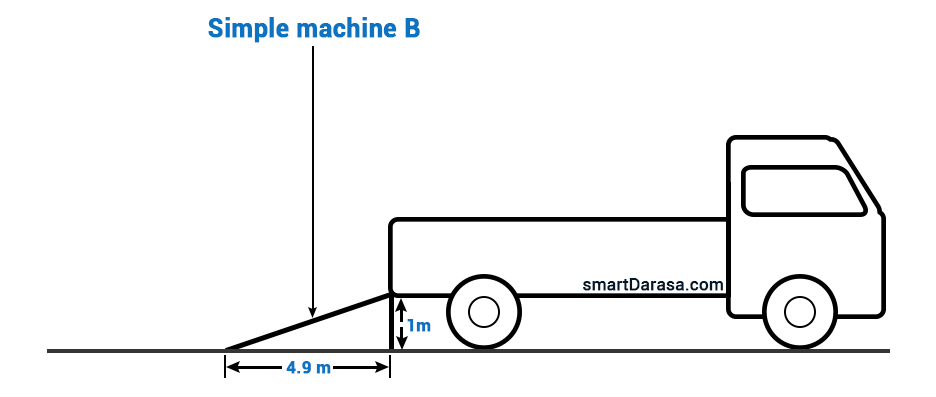• SECTION 01

(a) Figure 1 shows a simple machine B which has to be used to pull the packing case of 2000 N into the car by an effort of 500 N. Calculate the efficiency of machine B.Data:

Effort = 500N,

Distance moved by effort =  (4.92 + 12)1/2  = 5.001m

Distance moved by load = 1m

Solution:

Efficient = Work Output/ Work Input = Wo/Wi

Work input involves work done by the effort was moving, in that case, Wi = Effort x Distance moved by effort = 500N x 5m = 2500Nm

Work output involves work done as the load was moving, in that case, Wo = Load x Distance moved by load = 2000N x 1m = 2000Nm

Efficient = Wo/Wi = 2000/2500 = 0.8

The efficient of machine is 0.8

• SECTION 02

(b) With the aid of a clearly labelled diagram, describe an experiment to investigate the relationship between the force acting on a body and the acceleration produced.A plot of acceleration against force

In order to determine the relationship between the Force and Acceleration experimental setup which involve a simple trolley, paper ticker paper and vibrating pin is set as diagram shows. When mass of the trolley increases the overall acceleration increases and resulting to variation on the paper ticker tape, the slot from the ticker tape are used to calculate the acceleration of an object, and when plotted against force they result to proportional relationship between force and acceleration.